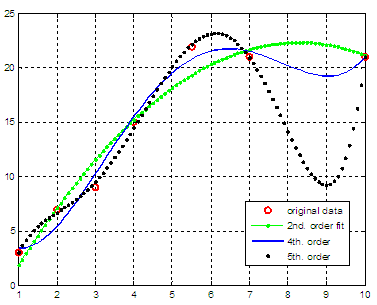Polynomial Regression – Least Square Fittings

This brief article will demonstrate how to work out polynomial regressions in Matlab (also known as polynomial least squares fittings). The idea is to find the polynomial function that properly fits a given set of data points.

 For this purpose, we’re going to use two useful built-in functions: polyfit (for fitting polynomial to data) and polyval (to evaluate polynomials). This is the simplest way to use these functions: p = polyfit(x, y, n) finds the coefficients of a polynomial p(x) of degree n that fits the data y best in a least-squares sense.

p is a row vector of length n + 1 containing the polynomial coefficients in descending powers, p(1)*x^n + p(2)*x^(n - 1) + ... + p(n)*x + p(n + 1).

y = polyval(p, x) returns the value of a polynomial p evaluated at x. p is a vector of length n + 1 whose elements are the coefficients of the polynomial in descending powers, y = p(1)*x^n + p(2)*x^(n - 1) + ... + p(n)*x + p(n + 1). If x is a matrix or vector, the polynomial is evaluated at all points in x.

Let’s say that we’re given these points

x = [1 2 3 4 5.5 7 10]
y = [3 7 9 15 22 21 21]

and want to explore fits of 2nd., 4th. and 5th. order. We could use the following code:

clear all, clc, close all, format compact

% define coordinates
x = [1 2 3 4 5.5 7 10];
y = [3 7 9 15 22 21 21];

% plot given data in red
plot(x, y, 'ro', 'linewidth', 2)
hold
on

% find polynomial fits of different orders
p2 = polyfit(x, y, 2)
p4 = polyfit(x, y, 4)
p5 = polyfit(x, y, 5)

% see interpolated values of fits
xc = 1 : .1 : 10;

% plot 2nd order polynomial
y2 = polyval(p2, xc);
plot(xc, y2,
'g.-')

% plot 4th order polynomial
y4 = polyval(p4, xc);
plot(xc, y4,
'linewidth', 2)

% plot 5th order polynomial
y5 = polyval(p5, xc);
plot(xc, y5,
'k.', 'linewidth', 2)
grid

legend('original data', '2nd. order fit', '4th. order', '5th. order')

The resulting fits are displayed in this figureThe coefficients found by Matlab are:

p2 =    -0.3863    6.3983   -4.1596
p4 =     0.0334   -0.7377    5.0158   -8.4137    7.5779
p5 =     0.0213   -0.5022    4.1330  -14.5708   25.3233  -11.3510

which, in other words, represent the following polynomials of  2nd., 4th. and 5th. order, respectively.

y2 = -0.3863x2 + 6.3983x - 4.1596
y4 =  0.0334x4 - 0.7377x3 + 5.0158x2 - 8.4137x + 7.5779
y5 =  0.0213x5 - 0.5022x4 + 4.1330x3 - 14.5708x2 + 25.3233x - 11.3510

You can see this video on how to do it even easier with the integrated Curve Fitting Tool within the Figure window.

From 'Polynomial Regression' to home

From 'Polynomial Regression' to Matlab Tutorials

 Top More on polynomials